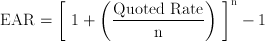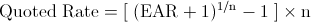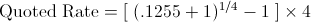# Business and Finance Math #3: Converting Effective Interest Rates (EAR) to Stated Interest Rates

Filed under Difficulty: Easy, Finance, HP 12c, TI BA II Plus.

In our previous blog post we introduced the concept of the effective annual rate (EAR), which is the true interest rate when compounding occurs more than one time per year. For example, 10% compounded semiannually is the same thing as 5% paid every 6 months, representing an annual interest rate of 10.25% per year.

This is an important concept to understand since the quoted interest rate may not be the interest rate you actually earn on your bank account (or pay on your credit card, car payment, or mortgage). While we converted a set of interest rates to the effective annual rate in our previous example to “standardize” the rates, this time we’re going to show you how to do the reverse process on your calculator: converting the EAR to a quoted rate.

Be sure to check our prior post on the effective annual interest rate for the background on the EAR.

## Interest Rate Math

In our first post, we introduced the formula for calculating the effective annual rate (EAR):This time, however, we want to calculate a hypothetical quoted rate for a given an EAR. By rearranging the EAR formula through some math magic, we can derive the mathematical formula for calculating the stated or quoted interest rate:Now let’s say we want to do the following:

Calculate the quoted rate compounded quarterly for an effective annual rate of 12.55%

Using our modified formula, we can input our effective annual rate and the number of periods we want to compound:Solving for the quoted interest rate, we find a solution of about 12% with quarterly compounding. Now let’s see how we can solve this type of problem on our financial calculator.

## Converting the EAR to a Quoted Interest Rate – TI BA II Plus Version

The TI BA II Plus makes it extremely easy to convert back and forth between an EAR and a stated or quoted interest rate. The steps to solve this problem are as follows:

1. Press the 2nd calculator button followed by the key. This selects the calculator’s ICONV function. You should see “EFF=” on the calculator screen. If not, use the or to navigate until you see EFF=
2. Type in 12.55, then press
3. Press the key, which should display “I/Y” on the calculator screen, then type in followed by since we want to calculate the quoted rate with quarterly compounding (4 times per year)
4. Now press one more time, followed by
5. You should see an interest rate of 11.99 on the calculator display

An EAR of 12.55% can also be written as 12% with quarterly compounding.

## Converting the EAR to a Quoted Interest Rate – HP 12c Version

To convert an EAR of 12.55% to a stated rate with quarterly compounding on the 12c, perform the following steps: